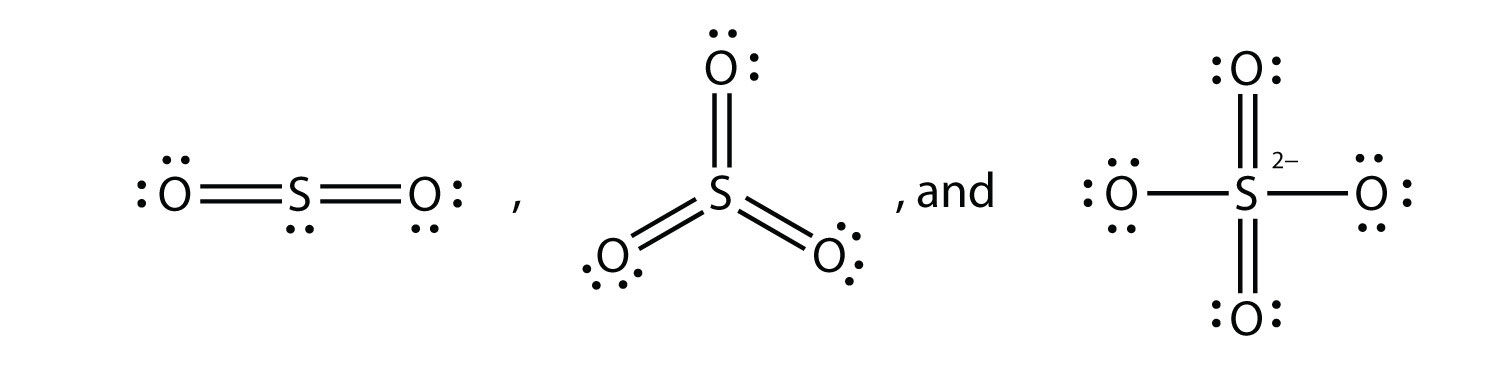QUESTION ANSWER 1. Explain why iron and copper have the same Lewis electron dot diagram when they have different numbers of electrons. 1. Iron has d electrons that typically are not shown on Lewis electron dot diagrams. 3. Based on the known trends, what ionic compound from the first column of the periodic table and the next-to-last column of the periodic table should have the highest lattice energy? 3. LiF 5. P2 is not a stable form of phosphorus, but if it were, what would be its likely Lewis electron dot diagram? 5. It would be like N2:7. What are the Lewis electron dot diagrams of SO2, SO3, and SO42−? 7.9. Which bond do you expect to be more polar—an O–H bond or an N–H bond? 9. an O–H bond 11. Use bond energies to estimate the energy change of this reaction. C3H8 + 5O2 → 3CO2 + 4H2O 11. −2,000 kJ 13. Ethylene (C2H4) has two central atoms. Determine the geometry around each central atom and the shape of the overall molecule. 13. trigonal planar about both central C atoms